# bmstdr: Bayesian Modeling of Spatio-Temporal Data with R

University of Southampton
S.K.Sahu@soton.ac.uk

#### 2022-04-05

Abstract

This is a vignette for the R package bmstdr. The package facilitates Bayesian modeling of both point reference and areal unit data with or without temporal replications. Three main functions in the package: Bspatial for spatial only point referene data, Bsptime for spatio-temporal point reference data and Bcartime for areal unit data, which may also vary in time, perform the main modeling and validation tasks. Computations and inference in a Bayesian modeling framework are done using popular R software packages such as spBayes, spTimer, spTDyn, CARBayes, CARBayesST and also code written using computing platforms INLA and rstan. Point reference data are modeled using the Gaussian error distribution only but a top level generalized linear model is used for areal data modeling. The user of bmstdr is afforded the flexibility to choose an appropriate package and is also free to name the rows of their input data frame for validation purposes. The package incorporates a range of prior distributions allowable in the nominated packages with default hyperparameter values. The package allows quick comparison of models using both model choice criteria, such as DIC and WAIC, and facilitates K-fold cross-validation without much programming effort. Familiar diagnostic plots and model fit exploration using the S3 methods such as summary, residuals and plot are included so that a beginner user confident in model fitting using the base R function lm can quickly learn to analyzing data by fitting a range of appropriate spatial and spatio-temporal models. This vignette illustrates the package using five built-in data sets. Three of these are on point reference data on air pollution and temperature at the deep ocean and the other two are areal unit data sets on Covid-19 mortality in England.

# 1 Introduction

Model-centric analysis of spatial and spatio-temporal data is essential in many applied areas of research such as atmospheric sciences, climatology, ecology, environmental health and oceanography. Such diversity in application areas is being serviced by the rich diversity of R contributed packages listed in the abstract and many others, see the CRAN Task Views: Handling and Analyzing Spatio-Temporal Data and Analysis of Spatial Data. Moreover, there are a number of packages and text books discussing handling of spatial and spatio-temporal data. For example, see the references Pebesma and Bivand (2005), Bivand, Pebesma, and Gomez-Rubio (2013), Pebesma (2012), Millo and Piras (2012), Banerjee, Carlin, and Gelfand (2015), Wikle, Zammit-Mangion, and Cressie (2019) and Sahu (2022). The diversity in packages, however, is also a source of challenge for an applied scientist who is also interested in exploring solutions offered by other models from rival packages. The challenge comes from the essential requirement to learn the package specific commands and setting up of the prior distributions that are to be used for the applied problem at hand.

The current package bmstdr sets out to help researchers in applied sciences model a large variety of spatial and spatio-temporal data using a multiplicity of packages but by using only three commands with different options. Point reference spatial data, where each observation comes with a single geo-coded location reference such as a latitude-longitude pair, can be analyzed by fitting several spatial and spatio-temporal models using R software packages such as spBayes (Finley, Banerjee, and Gelfand 2015), spTimer (Bakar and Sahu 2015), INLA (Rue, Martino, and Chopin 2009), rstan (Stan Development Team 2020), and spTDyn (Bakar, Kokic, and Jin 2016).
A particular package is chosen with the package= option to the bmstdr model fitting routines Bspatial for point reference spatial only data and Bsptime for spatio-temporal data. In each of these cases a Bayesian linear model, which can be fitted with the option package="none" provides a base line for model comparison purposes. For areal unit data modeling the bmstdr function Bcartime provides opportunities for model fitting using three packages: CARBayes (Lee 2021), CARBayesST (Lee, Rushworth, and Napier 2018) and INLA (Blangiardo and Cameletti 2015). Here also a base line Bayesian generalized linear model for independent data, fitted using CARBayes, is included for model comparison purposes.

Models fitted using bmstdr can be validated using the optional argument validrows, which can be a vector of row numbers of the model fitting data frame, to any of the three model fitting functions. The package then automatically sets aside the nominated data rows as specified by the validrows argument and use the remaining data rows for model fitting. Inclusion of this argument also automatically triggers calculation of four popular model validation statistics: root mean square error, mean absolute error, continuous ranked probability score (Gneiting and Raftery 2007) and coverage percentage. While performing validation the package also produces a scatter plot of predictions against observations with further options controlling the behavior of this plot.

The generality of the platforms INLA and rstan allows tremendous flexibility in modeling and validation. However, bespoke code must be written to implement each different model. The current version of bmstdr provides a limited number of models which can be fitted using INLA and rstan. For point reference spatial only data a marginal model, after integrating the spatial random effects, with a known exponential correlation function is implemented using rstan and for spatio-temporal point reference data a marginal model has also been implemented using this package. A marginal model has also been implemented in a separate bmstdr model fitting function called Bmoving_sptime which facilitates modeling of point reference temporal data from moving sensors such as Argo floats in the oceans, see Section 3.11. INLA based models use a discretized Gaussian Markov random field with penalized complexity prior distribution (Fuglstad et al. 2018).

For areal unit data modeling the bmstdr function Bcartime provides opportunities for selected model fitting using CARBayes (Lee 2021), and CARBayesST (Lee, Rushworth, and Napier 2018) CARBayesST(Lee, Rushworth, and Napier 2018) and also INLA as illustrated by Blangiardo and Cameletti (2015). Here also a base line Bayesian generalized linear model for independent data, fitted using CARBayes, is included for model comparison purposes. The INLA based models can fit the celebrated Besag, York, and Mollié (1991) model and the Leroux model (Leroux, Lei, and Breslow 2000).

Models fitted using bmstdr can be validated using the optional argument validrows, which can be a vector of row numbers of the model fitting data frame, to any of the three model fitting functions. The package then automatically sets aside the nominated data rows as specified by the validrows argument and use the remaining data rows for model fitting. Inclusion of this argument also automatically triggers calculation of four popular model validation statistics: root mean square error, mean absolute error, continuous ranked probability score (Gneiting and Raftery 2007) and coverage percentage. While performing validation, the package also produces a scatter plot of predictions against observations with further options controlling the behavior of this plot.

The remainder of this vignette is organized as follows. Section 2 illustrates point reference spatial data modeling with Gaussian error distribution. Section 3 discusses Gaussian models for point reference spatio-temporal data. Area data are modeled in Section 4 where Section 4.3 illustrates models for static areal unit data and Section 4.4 considers areal temporal data. Some summary remarks are provided in Section 5.

# 2 Point reference spatial data modeling

## 2.1 Illustration data set nyspatial

To illustrate point reference spatio-temporal data modeling we use the nyspatial data set included in the package. This data set has 28 rows and 9 columns containing average ground level ozone air pollution data from 28 sites in the state of New York. The averages are taken over the 62 days in July and August 2006. The full spatio-temporal data set from 28 sites for 62 days is used to illustrate spatio-temporal modeling, see Section 3.1. Figure 2.1 represents a map of the state of New York together with the 28 monitoring locations where the three sites 1, 5 and 10 have been identified.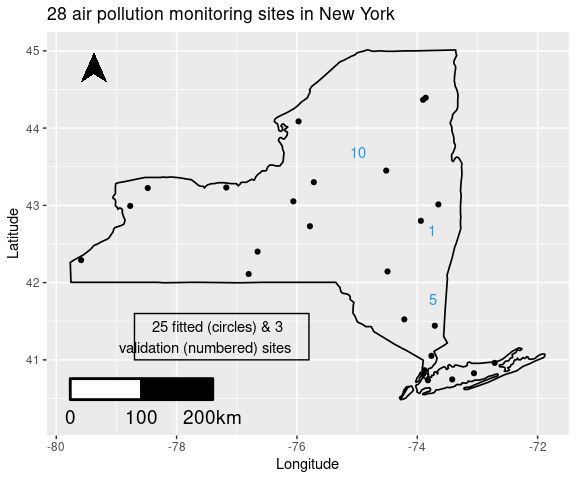Figure 2.1: 25 fitted sites and 3 validation sites (numbered) in New York

For regression modeling purposes, the response variable is yo3 and the three important covariates are maximum temperature: xmaxtemp in degree Celsius, wind speed: xwdsp in nautical miles and percentage average relative humidity: xrh. This data set is included in the package and further information regarding this can be obtained from the help file ?nyspatial.

## 2.2 The Bspatial function for fitting spatial regression models

The bmstdr package includes the function Bspatial for fitting regression models to point referenced spatial data. The arguments to this function has been documented in the help file which can be viewed by issuing the R command ?Bspatial. The package manual also contains the full documentation. The discussion below highlights the main features of this model fitting function.

Besides the usual data and formula the argument scale.transform can take one of three possible values: NONE, SQRT and LOG. This argument defines the on the fly transformation for the response variable which appears on the left hand side of the formula.

Default values of the arguments prior.beta0, prior.M and prior.sigma2 defining the prior distributions for $$\mathbf{\beta}$$ and $$1/\sigma^2_{\epsilon}$$ are provided.

The options model="lm" and model="spat" are respectively used for fitting and analysis using the independent spatial regression model with exponential correlation function. If the latter regression model is to be fitted, the function requires three additional arguments, coordtype, coords and phi. The coords argument provides the coordinates of the data locations. The type of these coordinates, specified by the coordtype argument, taking one of three possible values: utm, lonlat and plain determines various aspects of distance calculation and hence model fitting. The default for this argument is utm when it is expected that the coordinates are supplied in units of meter. The coords argument provides the actual coordinate values and this argument can be supplied as a vector of size two identifying the two column numbers of the data frame to take as coordinates. Or this argument can be given as a matrix of number of sites by 2 providing the coordinates of all the data locations.

The parameter phi determines the rate of decay of the spatial correlation for the assumed exponential covariance function. The default value, if not provided, is taken to be 3 over the maximum distance between the data locations so that the effective range is the maximum distance.

The argument package chooses one package to fit the spatial model from among four possible choices. The default option none is used to fit the independent linear regression model and the also the spatial regression model without the nugget effect when the parameter phi is assumed to be known. The three other options are spBayes, stan and inla. Each of these options use the corresponding R packages for model fitting. The exact form of the models in each case is documented in Chapter 6 of the book Sahu (2022).

Calculation of model choice statistics is triggered by the option mchoice=T. In this case the DIC, WAIC and PMCC values are calculated.

An optional vector argument validrows providing the row numbers of the supplied data frame for model validation can also be given. The model choice statistics are calculated on the opted scale but model validations and their uncertainties are calculated on the original scale of the response for ease of interpretation. This strategy of a possible transformed modeling scale but predictions on the original scale is adopted throughout the package.

There are other arguments of Bspatial, e.g. verbose, which control various aspects of model fitting and return values. Some of these other arguments are only relevant for specifying prior distributions and performing specific tasks as we will see throughout the remainder of this section.

The return value of Bspatial is a list of class bmstdr providing parameter estimates, and if requested model choice statistics and validation predictions and statistics. The S3methods print, plot, summary, fitted, and residuals have been implemented for objects of the bmstdr class. Thus the user can give the commands such as summary(M1) and plot(M1) where M1 is the model fitted object .

## 2.3 Fitting independent error regression models

The bmstdr package allows us to fit the base linear regression model given by: $\begin{equation} Y_i = \beta_1 x_{i1} + \ldots + \beta_p x_{ip} + \epsilon_i, i=1, \ldots, n \tag{2.1} \end{equation}$ where $$\beta_1, \ldots, \beta_p$$ are unknown regression coefficients and $$\epsilon_i$$ is the error term that we assume to follow the normal distribution with mean zero and variance $$\sigma^2_{\epsilon}$$. The usual linear model assumes the errors $$\epsilon_i$$ to be independent for $$i=1, \ldots, n$$. With the suitable default assumptions regarding the prior distributions we can fit the above model (2.1) by using the following command:

M1 <- Bspatial(formula=yo3~xmaxtemp+xwdsp+xrh, data=nyspatial, mchoice=T)

## 2.4 Fitting linear models with spatial error distribution

The independent linear regression model (2.1) is now extended to have spatially colored covariance matrix $$\sigma^{2}_{\epsilon}H$$ where $$H$$ is a known correlation matrix of the error vector $$\mathbf{\epsilon}$$, i.e. $$H_{ij}=\mbox{Cor}(\epsilon_i, \epsilon_j)$$ for $$i, j=1, \ldots,n$$, $\begin{equation} {\bf Y} \sim N_n \left(X{\mathbf \beta}, \sigma^{2}_{\epsilon} H\right) \tag{2.2} \end{equation}$ Assuming the exponential correlation function, i.e., $$H_{ij} = \exp(-\phi d_{ij})$$ where $$d_{ij}$$ is the distance between locations $${\bf s}_i$$ and $${\bf s}_j$$ we can fit the model (2.2) by issuing the command:

M2 <- Bspatial(model="spat", formula=yo3~xmaxtemp+xwdsp+xrh, data=nyspatial,
coordtype="utm", coords=4:5, phi=0.4, mchoice=T)

We discuss the choice of the fixed value of the spatial decay parameter $$\phi=0.4$$ in M2. We use cross-validation methods to find an optimal value for $$\phi$$. We take a grid of values for $$\phi$$ and calculate a cross-validation error statistics, e.g. root mean square-error (rmse), for each value of $$\phi$$ in the grid. The optimal $$\phi$$ is the one that minimizes the statistics.

To perform the grid search a simple R function, phichoice_sp is provided. The documentation of this function explains how to set the arguments. For example, the following commands work:

asave <- phichoice_sp(formula=yo3~xmaxtemp+xwdsp+xrh, data=nyspatial, coordtype="utm", coords=4:5, phis=seq(from=0.1, to=1, by=0.1), scale.transform="NONE", s=c(8,11,12,14,18,21,24,28), N=2000, burn.in=1000)
asave

For the nyspatial data example 0.4 turns out to be the optimal value for $$\phi$$.

## 2.5 Fitting spatial models with nugget effect

A general spatial model with nugget effect is written as: $\begin{equation} Y({\bf s}_i) = {\bf x}'({\bf s}_i) \mathbf{\beta} + w({\bf s}_i) + \epsilon( {\bf s}_i) \tag{2.3} \end{equation}$ for all $$i=1, \ldots, n$$. In the above equation, the pure error term $$\epsilon({\bf s}_i)$$ is assumed to follow the independent zero mean normal distribution with variance $$\sigma^2_{\epsilon}$$, called the nugget effect, for all $$i=1. \ldots, n$$. The stochastic process $$w({\bf s})$$ is assumed to follow a zero mean Gaussian Process with the exponential covariance function, see Sahu (2022) for more details.

The un-observed random variables $$w({\bf s}_i)$$, $$i=1, \ldots, n$$, also known as the spatial random effects can be integrated out to arrive at the marginal model \begin{align} {\bf Y} & \sim N\left(X{\mathbf \beta}, \sigma^2_{\epsilon} \, I + \sigma^2_w S_w \right), \tag{2.4} \end{align} where the matrix $$S_w$$ is determined using the exponential correlation function. This marginal model is fitted using any of the three packages mentioned above. The code for this model fitting is very similar to the one for fitting M2 above; the only important change is in the package= argument as noted below.

M3 <- Bspatial(package="spBayes", formula=yo3~xmaxtemp+xwdsp+xrh, data=nyspatial,
coordtype="utm", coords=4:5, prior.phi=c(0.005, 2), mchoice=T)
M4 <- Bspatial(package="stan", formula=yo3~xmaxtemp+xwdsp+xrh, data=nyspatial,
coordtype="utm", coords=4:5,phi=0.4, mchoice=T)
M5  <- Bspatial(package="inla",formula=yo3~xmaxtemp+xwdsp+xrh, data=nyspatial,
coordtype="utm", coords=4:5, mchoice=T)

Model fitting is very fast except for M4 with the stan package. The model run for M4 takes about 20 minutes on a fast personal computer. These run produces the values of various Bayesian model choice criteria shown in Table 2.2.

Criteria pdic pdicalt dic dicalt pwaic1 M0 M1 M2 M3 M4 M5 2.07 4.99 4.98 5.17 5.31 4.17 13.58 5.17 5.16 7.83 6.46 169.20 158.36 158.06 158.68 158.75 157.23 192.22 158.72 158.41 163.99 161.04 1.82 5.20 4.93 4.88 4.93 4.73 2.52 6.32 5.91 6.77 5.96 168.95 158.57 157.51 158.70 157.92 158.46 170.35 160.82 159.47 162.48 159.99 591.82 327.98 330.08 323.56 316.67 334.03 577.13 351.52 346.73 396.63 394.86 39.17 1168.95 679.50 676.82 720.18 711.52 373.19

Mathematical expressions for all the quantities in the above table are provided in Sahu (2022). Here M0 is the intercept only model for which the results are obtained using the following bmstdr command,

Bmchoice(case="MC.sigma2.unknown", y=ydata).

The implementation using inla does not calculate the alternative values of the DIC and WAIC.

## 2.6 Illustrating the model validation statistics

The model fitting function Bspatial also calculates the values of four validation statistics: - root mean square-error (rmse), - mean absolute error (mae), - continuous ranked probability score (crps) and - coverage (cvg) if an additional argument validrows containing the row numbers of the supplied data frame to be validated is provided.

Data from eight validation sites 8, 11, 12, 14, 18, 21, 24 and 28 are set aside and model fitting is performed using the data from the remaining 20 sites.

The bmstdr command for performing validation needs an additional argument validrows which are the row numbers of the supplied data frame which should be used for validation.

s <- c(8,11,12,14,18,21,24,28)
f1 <- yo3~xmaxtemp+xwdsp+xrh
M1.v <-  Bspatial(package="none", model="lm", formula=f1,
data=nyspatial, validrows=s)
M2.v <- Bspatial(package="none", model="spat", formula=f1,
data=nyspatial,   coordtype="utm", coords=4:5,phi=0.4,  validrows=s)
M3.v <-  Bspatial(package="spBayes", prior.phi=c(0.005, 2), formula=f1,
data=nyspatial,   coordtype="utm", coords=4:5, validrows=s)
M4.v  <- Bspatial(package="stan",formula=f1,
data=nyspatial,   coordtype="utm", coords=4:5,phi=0.4 , validrows=s)
M5.v  <- Bspatial(package="inla", formula=f1, data=nyspatial,
coordtype="utm", coords=4:5, validrows=s) 

Table 2.4 presents the validation statistics for all five models. Coverage is 100% for all five models and the validation performances are comparable. Model M4 with $$\phi=0.4$$ can be used as the best model if it is imperative that one must be chosen using the rmse criterion.

 Criteria rmse mae crps M1 M2 M3 M4 M5 2.447 2.400 2.428 2.422 2.380 2.135 2.015 2.043 2.054 1.971 2.891 2.885 2.891 2.037 2.137

To illustrate $$K$$-fold cross-validation, the 28 observations in the nyspatial data set are randomly assigned to $$K=4$$ groups of equal size.

set.seed(44)
x <- runif(n=28)
u <- order(x)
s1 <- u[1:7]
s2 <- u[8:14]
s3 <- u[15:21]
s4 <- u[22:28]

Now the M2.v command is called four times with the validrows argument taking values s1, ... s4. Table 2.6 presents the 4-fold cross-validation statistics for M2 only. It shows a wide variability in performance with a low coverage of 57.14% for Fold 3.

Criteria rmse mae crps Fold1 Fold2 Fold3 Fold4 2.441 5.005 5.865 2.508 1.789 3.545 5.462 2.145 2.085 2.077 1.228 2.072 100.000 85.714 57.143 100.000

A validation plot is automatically drawn each time a validation is performed. Below, we include the validation plot for fold-3 only.

M2.v3 <- Bspatial(model="spat", formula=yo3~xmaxtemp+xwdsp+xrh, data=nyspatial,
coordtype="utm", coords=4:5, validrows= s3, phi=0.4, verbose = FALSE)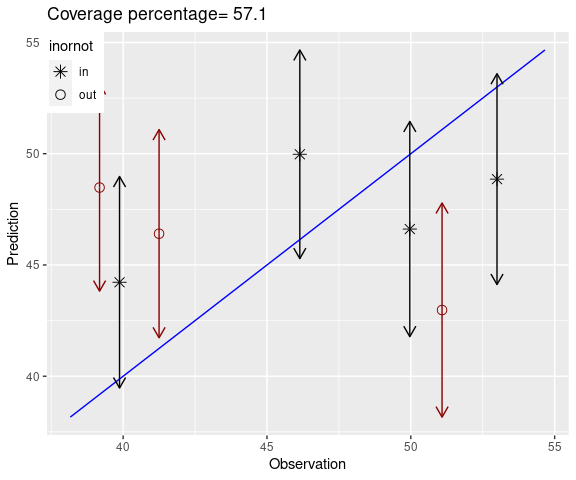Figure 2.2: Prediction against observation plot with the prediction intervals included. The in/out’ symbol in the plot indicates whether or not a prediction interval includes the 45 degree line.

In this particular instance four of the seven validation observations are over-predicted. The above figure shows low coverage and high rmse. However, these statistics are based on data from seven validation sites only and as a result these may have large variability explaining the differences in the $$K$$-fold validation results.

The above validation plot has been drawn using the bmstdr command obs_v_pred_plot. This validation plot may be drawn without the line segments, which is recommended when there are a large number of validation observations. The plot may also use the mean values of the predictions instead of the default median values. The documentation of the function explains how to do this. For example, having the fitted object M2.v3, we may issue the commands:

names(M2.v3)
psums <- get_validation_summaries(M2.v3$valpreds) names(psums) a <- obs_v_pred_plot(yobs=M2.v3$yobs_preds$yo3, predsums=psums, segments=FALSE, summarystat = "mean" ) # 3 Point reference spatio-temporal data modeling ## 3.1 Illustration data set nysptime To illustrate point reference spatio-temporal data modeling we use the nysptime data set included in the package. This is a spatio-temporal version of the data set nyspatial introduced in Section 2.1. This data set, taken from Sahu and Bakar (2012), has 1736 rows and 12 columns containing ground level ozone air pollution data from 28 sites in the state of New York for the 62 days in July and August 2006. For regression modeling purposes, the response variable is y8hrmax and the three important covariates are maximum temperature: xmaxtemp in degree Celsius, wind speed: xwdsp in nautical miles and percentage average relative humidity: xrh. ## 3.2 The Bsptime function for fitting spatio-temporal models In this section we extend the spatial model (2.3) to the following spatio-temporal model. $\begin{equation} Y({\bf s}_i, t) = {\bf x}'({\bf s}_i, t) \mathbf{\beta} + w({\bf s}_i, t) + \epsilon( {\bf s}_i, t) \tag{3.1} \end{equation}$ for $$i=1, \ldots, n$$ and $$t=1, \ldots, T.$$ Different distribution specifications for the spatio-temporal random effects $$w({\bf s}_i, t)$$ and the observational errors $$\epsilon({\bf s}_i, t)$$ give rise to different models. Variations of these models have been described in Sahu (2022). The bmstdr function Bsptime has been developed to fit these models. Similar to the Bspatial function, the Bsptime function takes a formula and a data argument. It is important to note that the Bsptime function always assumes that the data frame is first sorted by space and then time within each site in space. Note that missing covariate values are not permitted. The arguments defining the scale, scale.transform, and the hyper parameters of the prior distribution for the regression coefficients $${\mathbf \beta}$$ and the variance parameters are also similar to the corresponding ones in the spatial model fitting case with Bspatial. Other important arguments are described below. The arguments coordtype, coords, and validrows are also similarly defined as before. However, note that when the separable model is fitted the validrows argument must include all the rows of time points for each site to be validated. The package argument can take one of six values: spBayes, stan, inla, spTimer, sptDyn and none with none being the default. Fittings using each of these package options are illustrated in the sections below. Only a limited number of models, specified by the model argument, can be fitted with each of these six choices. The model argument is described below. In case the package is none, the model can either be lm or seperable. The lm option is for an independent error regression model while the other option fits a separable spatio-temporal model without any nugget effect. The separable model fitting method cannot handle missing data. All missing data points in the response variable will be replaced by the grand mean of the available observations. When the package option is one of the five named packages the model argument is passed to the chosen package. For fitting a separable model Bsptime requires specification of two decay parameters $$\phi_s$$ and $$\phi_t$$. If these are not specified then values are chosen which correspond to the effective ranges as the maximum distance in space and length in time. There are numerous other package specific arguments that define the prior distributions and many important behavioral aspects of the selected package. Those are not described here. Instead the user is directed to the documentation ?Bsptime and also the vignettes of the individual packages. With the default value of package="none" the independent error regression model M1 and the separable model M2 are fitted using the commands: f2 <- y8hrmax~xmaxtemp+xwdsp+xrh M1 <- Bsptime(model="lm", formula=f2, data=nysptime, scale.transform = "SQRT") M2 <- Bsptime(model="separable", formula=f2, data=nysptime, scale.transform = "SQRT", coordtype="utm", coords=4:5) The fitted model objects M1 and M2 are of class bmstdr and these can be explored using the S3 methods print, plot, summary and residuals. To explore the model fitted object issue the command names(M2). Here we explore the residuals by issuing the command: a <- residuals(M2) #> #> Note that the residuals are provided on the transformed scale. Please see the scale.transform argument. #> #> Summary of the residuals #> Min. 1st Qu. Median Mean 3rd Qu. Max. NA's #> -3.13367 -0.98683 -0.49008 -0.49402 0.02336 2.11039 24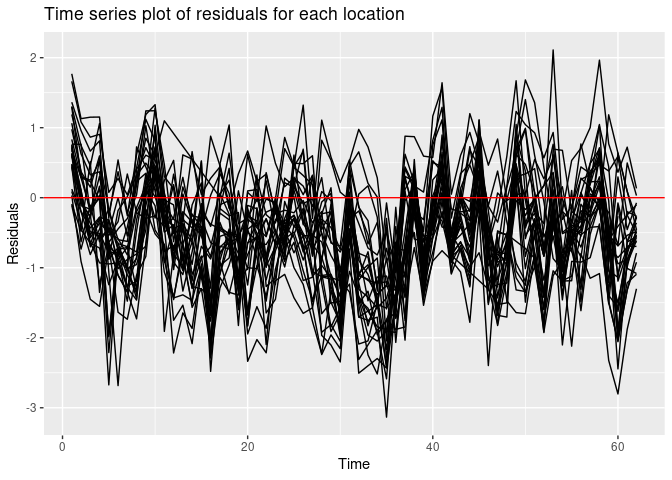Figure 3.1: A multiple time series plot of residuals This command renders a multiple time series plot of the residuals. However, the same command a <- residuals(M1) will not draw the residual plot since the independent error regression model is not aware of the temporal structure of the data. In this case it is possible to modify the command to a <- residuals(M1, numbers=list(sn=28, tn=62)) to have the desired result, see ?residuals.bmstdr. ## 3.3 Exploring the separable model The above command for fitting M2 does not specify the values of the spatial and temporal decay parameters $$\phi_s$$ and $$\phi_t$$. The adopted values of these parameters are printed by the command: M2$phi.s; M2$phi.t These values are approximately 0.005 and 0.048 which correspond to the spatial range (the value of distance by which spatial correlation dies down) of 591 kilometers and the temporal range of 62 days which are the maximum possible values for the spatial and temporal domains of the data. Optimal values of $$\phi_s$$ and $$\phi_t$$ can be determined by performing a grid search as in the case spatial only model fitting with Bspatial. The bmstdr package contains a function called phichoice which does this grid search specifically for the nysptime data set using the eight validation sites noted earlier. The command is: asave <- phichoicep(formula=y8hrmax ~ xmaxtemp+xwdsp+xrh, data=nysptime, coordtype="utm", coords=4:5, scale.transform = "SQRT", phis=c(0.001, 0.005, 0.025, 0.125, 0.625), phit=c(0.05, 0.25, 1.25, 6.25), valids=c(8,11,12,14,18,21,24,28), N=2000, burn.in=1000) The optimal values in this case turns out to be $$\phi_s=0.005$$ and $$\phi_t=0.05$$ approximately. We now explore model validation for the separable model using the three sites chosen previously. valids <- c(1, 5, 10) vrows <- which(nysptime$s.index%in% valids)
M2.1 <- Bsptime(model="separable",  formula=f2, data=nysptime,
validrows=vrows, coordtype="utm", coords=4:5,
phi.s=0.005, phi.t=0.05, scale.transform = "SQRT")
summary(M2.1)
#> Call:
#> Bsptime(formula = f2, data = nysptime, model = "separable", coordtype = "utm",
#>     coords = 4:5, validrows = vrows, scale.transform = "SQRT",
#>     phi.s = 0.005, phi.t = 0.05)
#> ##
#> # Total time taken:: 3.08  - Sec.
#> Model formula
#> y8hrmax ~ xmaxtemp + xwdsp + xrh
#>
#>
#> Parameter Estimates:
#>               mean    sd   2.5%  97.5%
#> (Intercept)  0.319 1.775 -3.161  3.799
#> xmaxtemp     0.256 0.038  0.182  0.330
#> xwdsp        0.010 0.036 -0.062  0.081
#> xrh         -0.024 0.113 -0.246  0.198
#> sigma2      12.442 0.447 11.597 13.348
#>
#> Validation Statistics:
#>    rmse     mae    crps     cvg
#>   6.895   5.583  11.868 100.000

The fitted model M2.1 can be passed to the plot function that may draw several plots depending on the contents of the model fitted object. For example, this always draws a residuals against fitted values plot. The plot command for objects of class bmstdr can take additional arguments such as segments = FALSE which will provide a plot of the predicted values against observations without the line segments.

## 3.4 Spatio-temporal model fitting with the spTimer package

The spTimer package Bakar and Sahu (2015) can be used to fit, predict and forecast using various spatio-temporal models. This package also offers a great deal of flexibility in data modeling since it can model segmented time series data and also mixture of discrete and continuous data such as precipitation. The bmstdr function Bsptime can implement these models by invoking the package="spTimer" option. For example:

M3 <- Bsptime(package="spTimer", formula=f2, data=nysptime, n.report=5,
coordtype="utm", coords=4:5, scale.transform = "SQRT")

As before, the fitted model object M3 can be explored using the print, summary, plot and residuals commands. The plot command will draw the MCMC trace and density plots for each model parameter. The output plots of these commands are omitted from this document for brevity. Instead, we show validation performance at three selected sites: 1, 5, and 10 as shown in the map.

We randomly select 31 time points for validation at the three selected sites and identify the validation rows by using the following commands.

set.seed(44)
tn <- 62
sn <- 28
valids <- c(1, 5, 10)
validt <- sort(sample(1:tn, size=31))
vrows <- getvalidrows(sn=sn, tn=tn, valids=valids, validt=validt)

The getvalidrows command is included in the databmstdr library. Now we use the spTimer package to fit and validate the model.

M31 <- Bsptime(package="spTimer",formula=f2, data=nysptime,
coordtype="utm", coords=4:5,
validrows=vrows, model="GP", report=5,
mchoice=F, scale.transform = "NONE")

For ease of illustration we have chosen scale.transform = "NONE". More complicated additional coding will be required if a different scale is chosen.

We illustrate a plot of the observations, fitted values and the validation predictions and their uncertainty intervals as plotted in Figure 11.13 in the book Banerjee, Carlin, and Gelfand (2015). The databmstdr package includes the function fig11.13.plot to generate this plot. The arguments required for this function are prepared as follows. The spTimer package does not provide the 95% intervals for the fitted values nor does it provide the MCMC samples of the fitted values which can be used to construct the 95% intervals. Hence, in the code below we use a normal approximation to obtain the uncertainty intervals. Now we first organize the data for plotting.

modfit <- M31$fit fitall <- data.frame(modfit$fitted)
fitall$s.index <- rep(1:sn, each=tn) library(spTimer) #> #> ## spTimer version: 3.3.1 vdat <- spT.subset(data=nysptime, var.name=c("s.index"), s=valids) fitvalid <- spT.subset(data=fitall, var.name=c("s.index"), s=valids) fitvalid$low <- fitvalid$Mean - 1.96 * fitvalid$SD
fitvalid$up <- fitvalid$Mean + 1.96 * fitvalid$SD fitvalid$yobs <- vdat$y8hrmax yobs <- matrix(fitvalid$yobs, byrow=T, ncol=tn)
y.valids.low <- matrix(fitvalid$low, byrow=T, ncol=tn) y.valids.med <- matrix(fitvalid$Mean, byrow=T, ncol=tn)
y.valids.up <- matrix(fitvalid$up, byrow=T, ncol=tn) Now we call the fig11.13.plot function to render the plot for each site. p1 <- fig11.13.plot(yobs[1, ], y.valids.low[1, ], y.valids.med[1, ], y.valids.up[1, ], misst=validt) p1 <- p1 + ggtitle("Validation for Site 1") p2 <- fig11.13.plot(yobs[2, ], y.valids.low[2, ], y.valids.med[2, ], y.valids.up[2, ], misst=validt) p2 <- p2 + ggtitle("Validation for Site 5") p3 <- fig11.13.plot(yobs[3, ], y.valids.low[3, ], y.valids.med[3, ], y.valids.up[3, ], misst=validt) p3 <- p3 + ggtitle("Validation for Site 10") library(ggpubr) #> #> Attaching package: 'ggpubr' #> The following object is masked from 'package:huxtable': #> #> font ggarrange(p1, p2, p3, common.legend = TRUE, legend = "top", nrow = 3, ncol = 1)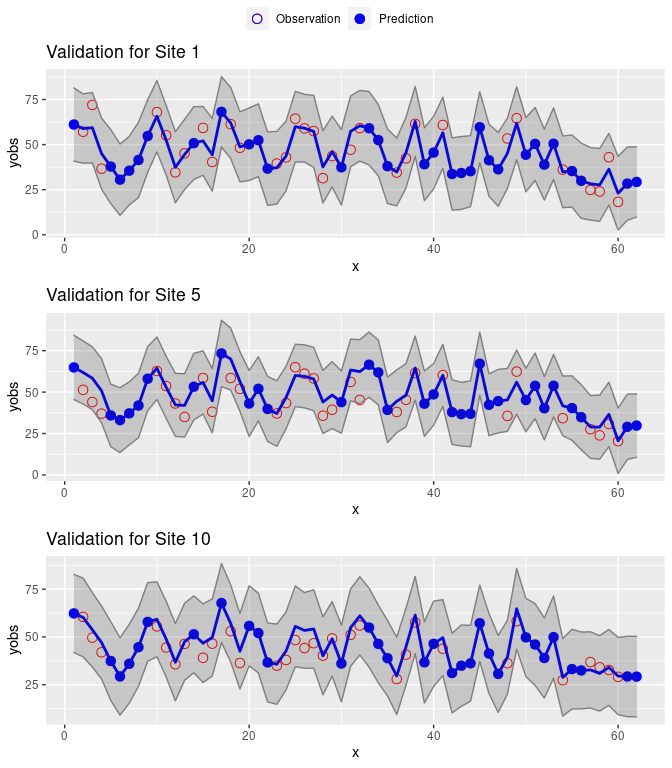Figure 3.2: Time series of observed and predicted values at three sites. This ability to fit and validate using user defined validation rows is an enhancement of the spTimer package since that only allows validation at all time points for any selected site. The original package does not allow selective predictions at a subset of time points. ### 3.4.1 Illustrating spatio-temporal predictions The spTimer package includes a prediction method function that can be used to predict at a large number locations. It does these predictions at all time points of the modeling data hence the covariates used in the model must be available for all prediction locations and at all time points. We illustrate the predictions using the fitted model M3. We only show the average predicted pollution map over the 62 days. To do the site-wise averaging we use the sitemeans function: sitemeans <- function(a, sn, tn=62) { u <- matrix(a, nrow=sn, ncol=tn, byrow=T) b <- apply(u, 1, mean) as.vector(b) } The bmstdr package includes the data set gridnysptime which contains the prediction data for 100 locations within the state of New York. Here is the code-chunk to perform prediction at these 100 locations and then averaging: post <- M3$fit
gpred <- predict(post, newdata=gridnysptime, newcoords=~Longitude+Latitude)
u <- gpred$pred.samples v <- apply(u, 2, sitemeans, sn=100) a <- get_parameter_estimates(t(v)) b <- data.frame(gridnyspatial[, 1:5], a)  The data frame b contains the location information and the prediction summaries at the 100 prediction sites. To draw the prediction map we also include the fitted values from the 28 data modeling sites. We extract the fitted values as follows: meanmat <- post$op
sig2eps <-  post$sig2ep sige <- sqrt(sig2eps) itmax <- ncol(meanmat) nT <- nrow(nysptime) sigemat <- matrix(rep(sige, each=nT), byrow=F, ncol=itmax) a <- matrix(rnorm(nT*itmax), nrow=nT, ncol=itmax) ypreds <- meanmat + a * sigemat ypreds <- (ypreds)^2 v <- apply(ypreds, 2, sitemeans, sn=28) a <- get_parameter_estimates(t(v)) fits <- data.frame(nyspatial[, 1:5], a) Finally we combine the predictions and the fitted values by the command: b <- rbind(b, fits) Now we can obtain the average pollution map by using the linear interpolation function interp in the akima library. Also we discard the interpolations outside the state of New York by using the function fnc.delete.map.XYZ included in the bmstdr package. coord <- nyspatial[, c("Longitude","Latitude")] library(akima) xo <- seq(from=min(coord$Longitude)-0.5, to = max(coord$Longitude)+0.8, length=200) yo <- seq(from=min(coord$Latitude)-0.25, to = max(coord$Latitude)+0.8, length=200) surf <- interp(b$Longitude, b$Latitude, b$mean,  xo=xo, yo=yo)
v <- fnc.delete.map.XYZ(xyz=surf)

interp1 <- data.frame(long = v$x, v$z )
names(interp1)[1:length(v$y)+1] <- v$y
library(tidyr)
interp1 <- gather(interp1,key = lat,value =Predicted,-long,convert = TRUE)
library(ggplot2)
nymap <- map_data(database="state",regions="new york")
mappath <- cbind(nymap$long, nymap$lat)
zr <- range(interp1$Predicted, na.rm=T) P <- ggplot() + geom_raster(data=interp1, aes(x = long, y = lat,fill = Predicted)) + geom_polygon(data=nymap, aes(x=long, y=lat, group=group), color="black", size = 0.6, fill=NA) + geom_point(data=coord, aes(x=Longitude,y=Latitude)) + stat_contour(data=na.omit(interp1), aes(x = long, y = lat,z = Predicted), colour = "black", binwidth =2) + scale_fill_gradientn(colours=colpalette, na.value="gray95", limits=zr) + theme(axis.text = element_blank(), axis.ticks = element_blank()) + ggsn::scalebar(data =interp1, dist = 100, location = "bottomleft", transform=T, dist_unit = "km", st.dist = .05, st.size = 5, height = .06, st.bottom=T, model="WGS84") + ggsn::north(data=interp1, location="topleft", symbol=12) + labs(x="Longitude", y = "Latitude", size=2.5)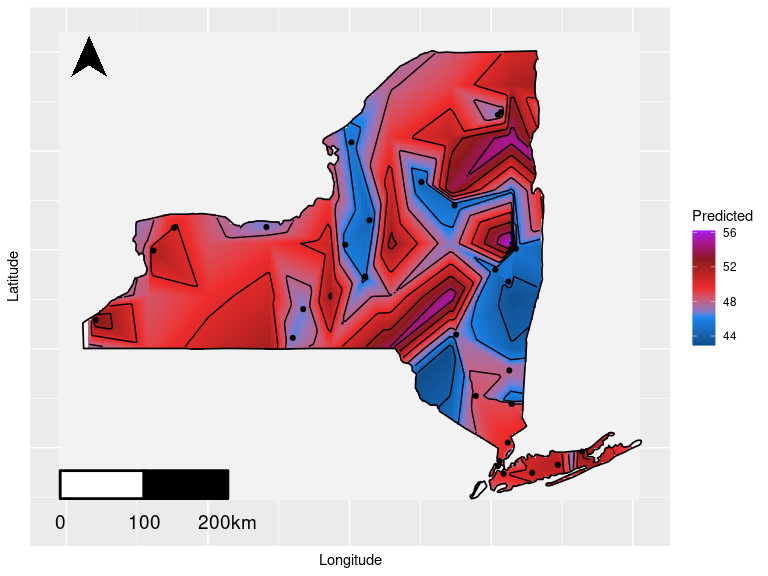Figure 3.3: Predicted map of average ozone air pollution in New York Similar methods are used to obtain a map of the standard deviations of the predictions which has been omitted for brevity. ## 3.5 Marginal model fitting with the rstan package The option package="stan" can be used to fit the only available model, which is a marginalized GP model, like (2.4) independently at each time point. Details for this model are provided in Chapter 7 of the book Sahu (2022). In this implementation the spatial decay parameter $$\phi$$ is sampled and its estimate is made available in the parameter estimates table. We illustrate model fitting by comparing the stan fitted model with the three previously fitted models. The commands for fitting all four models are: M1.c <- Bsptime(model="lm", formula=f2, data=nysptime, scale.transform = "SQRT", mchoice=T) M2.c <- Bsptime(model="separable", formula=f2, data=nysptime, coordtype="utm", coords=4:5, phi.s=0.005, phi.t=0.05, scale.transform = "SQRT", mchoice=T) M3.c <- Bsptime(package="spTimer", model="GP", formula=f2, data=nysptime, coordtype="utm", coords=4:5, scale.transform = "SQRT", mchoice=T, N=5000) M4.c <- Bsptime(package="stan",formula=f2, data=nysptime, coordtype="utm", coords=4:5, scale.transform = "SQRT", N=1500, burn.in=500, mchoice=T, verbose = F) The above commands have been executed to produce Table 3.2 of model choice criteria for the four models M1 to M4. Criteria pdic pdicalt dicorig M1 M2 M3 M4 4.98 4.98 78.65 30.36 4.94 4.95 841.96 31.22 3912.07 3214.55 3132.10 2695.11 3912.01 3214.50 4658.72 2696.83 4.85 14.39 48.53 9.05 4.87 14.58 132.90 10.04 3911.95 2448.00 2603.86 2088.15 3911.99 2448.38 2772.60 2090.12 963.24 286.08 216.75 328.74 965.58 240.38 873.84 361.95 1928.82 526.47 1090.59 690.69 Later we will investigate how these models perform by refitting the models by requesting to validate. ## 3.6 Autoregressive model fitting with spTimer and INLA Temporal auto regressive (AR) models can be fitted using both the spTimer and INLA package. The command for fitting the AR model using the spTimer package requires the additional option model="AR": M5 <- Bsptime(package="spTimer", model="AR", formula=f2, data=nysptime, coordtype="utm", coords=4:5, scale.transform = "SQRT", mchoice=T, validrows = vrows) To fit and validate with the AR model using the INLA package we issue the command: M6 <- Bsptime(package="inla", model="AR", formula=f2, data=nysptime, coordtype="utm", coords=4:5, scale.transform = "SQRT", mchoice=T, validrows=vrows) The AR models M5 and M6 produces the following model choice results in Table 3.4. Criteria spTimer INLA gof penalty pmcc 321.33 607.31 928.64 737.54 21.17 758.70 Parameter estimates from the two models are shown in Table 3.6. The INLA implemented model autoregressive model M6 has been fitted without an intercept as this has been seen to be better than spTimer model M5. Other parameter estimates are somewhat comparable but there are large differences also, see e.g., the estimates of the autoregressive parameter $$\rho$$ and the spatial decay parameter $$\phi$$. These differences are expected as the two packages use different model parameterisations and prior distributions. The current package bmstdr facilitates this sort of model comparison without much additional programming effort. Criteria (Intercept) xmaxtemp xwdsp xrh rho sig2eps sig2eta mean sd 2.5% 97.5% mean sd 2.5% 97.5% 1.437 0.540 0.383 2.513 0.091 0.015 0.060 0.122 0.241 0.003 0.235 0.247 0.031 0.023 -0.014 0.078 0.052 0.012 0.029 0.076 -0.191 0.060 -0.304 -0.074 -0.043 0.018 -0.079 -0.008 0.512 0.021 0.471 0.554 0.898 0.066 0.739 0.984 0.014 0.002 0.010 0.019 0.443 0.016 0.411 0.474 0.570 0.032 0.511 0.638 0.613 0.372 0.202 1.607 0.009 0.001 0.008 0.010 0.329 0.132 0.151 0.660 ## 3.7 Spatio-temporal dynamic models using the spTDyn package The spTDyn package can be used to fit dynamic models in both time and space. A spatial dynamic model allows the regression coefficient to vary in space. A temporal dynamic model allows the regression coefficient to have a dynamic equation in time. To invoke these dynamic models the regression variables are specified in the formula argument with the optional enclosures sp for spatially dynamic and tp for temporally dynamic. Here is an example where the model is specified to have spatially varying effects of maximum temperature and dynamic regression coefficients for wind speed. library(spTDyn) f3 <- y8hrmax~ xmaxtemp + sp(xmaxtemp)+ tp(xwdsp) + xrh M7 <- Bsptime(package="sptDyn", model="GP", formula=f3, data=nysptime, coordtype="utm", coords=4:5, scale.transform = "SQRT", n.report=2) The model fitting results can be examined by the S3 methods functions as before. Here we explore the spatially varying regression coefficients as follows: out <- M7$fit
dim(out$betasp) #>  28 1000 a <- out$betasp
u <- c(t(out$betasp)) sn <- nrow(a) itmax <- ncol(a) v <- rep(1:sn, each=itmax) d <- data.frame(site=as.factor(v), sp = u) p <- ggplot(data=d, aes(x=site, y=sp)) + geom_boxplot(outlier.colour="black", outlier.shape=1, outlier.size=0.5) + geom_abline(intercept=0, slope=0, color="blue") + labs(title= "Spatial effects of maximum temperature", x="Site", y = "Effects", size=2.5) p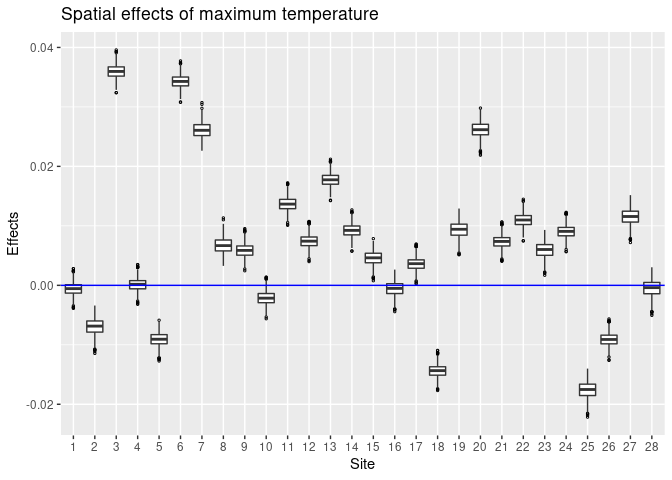Figure 3.4: Spatial effects of maximum temperature from model M7 Similarly, we provide a plot of the temporal effects parameter. b <- out$betatp
tn <- nrow(b)
itmax <- ncol(b)
tids <- 1:tn
stat <- apply(b[tids,], 1, quantile, prob=c(0.025,0.5,0.975))
tstat <- data.frame(tids, t(stat))
dimnames(tstat)[] <- c("Days", "low", "median", "up")
yr <- c(min(c(stat)),max(c(stat)))
p <- ggplot(data=tstat, aes(x=Days, y=median)) +
geom_point(size=3) +
ylim(yr) +
geom_segment(data=tstat, aes(x=Days, y=median, xend=Days, yend=low), linetype=1) +
geom_segment(data=tstat, aes(x=Days, y=median, xend=Days, yend=up), linetype=1) +
geom_abline(intercept=0, slope=0, col="blue") +
labs(title="Temporal effects of wind speed", x="Days", y="Temporal effects")
p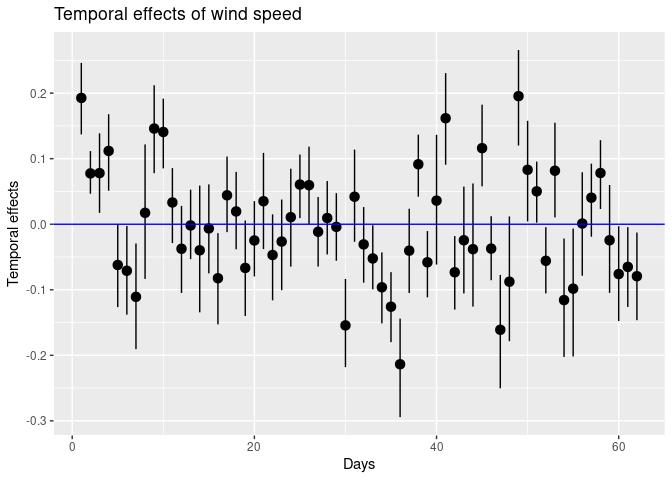Figure 3.5: Temporal effects of wind speed from model M7

## 3.8 Fitting dynamic models using spBayes

The package option package="spBayes" in the Bsptime function triggers model fitting by using a complex dynamic model due to Gelfand, Banerjee, and Gamerman (2005), see also Chapter 11 of the book Banerjee, Carlin, and Gelfand (2015). This model fitting is sensitive to the choice of the prior distributions and the options below leads to a reasonable model fit.

M8 <- Bsptime(package="spBayes",  formula=f2, data=nysptime,
prior.sigma2=c(2, 25),
prior.tau2 =c(2, 25),
prior.sigma.eta =c(2, 0.001),
coordtype="utm",
coords=4:5, scale.transform = "SQRT",
mchoice=T,  N=5000,  n.report=200)

The dynamic model parameters are extracted using the code below.

modfit <- M8$fit N <- 5000 burn.in <- 1000 tn <- 62 quant95 <- function(x){ quantile(x, prob=c(0.025, 0.5, 0.975)) } theta <- apply(modfit$p.theta.samples[burn.in:N,], 2, quant95)
sigma.sq <- theta[,grep("sigma.sq", colnames(theta))]
tau.sq <- theta[,grep("tau.sq", colnames(theta))]
phi <- theta[,grep("phi", colnames(theta))]

These extracted variance and the range ($$3/\phi$$) parameters are plotted using the ggplot` function. The details are omitted from this document. We also do not provide parameter plots for the regression parameters.